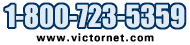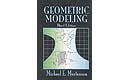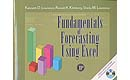Metalworking Tools and Supplies

Model # Qty

# Books on Mathematics

## Geometric Modeling, Third EditionClick for a Bigger Picture
Geometric Modeling, Third Edition
Michael E. Mortenson
452 pages, Illustrated, 7 x 10
Published: 2006

OVERVIEW
Completely updated to include the most recent developments in the field, the third edition like the two previous editions,
emphasizes clarity and thoroughness in the mathematical development of its subjects. It is written in a style that is free of
jargon of special applications, while integrating the three important functions of geometric modeling: to represent elementary
forms (curves, surfaces, and solids), to shape and assemble these into complex forms, and to determine geometric properties
and relationships. With hundreds of illustrations, this unique book appeals to the readers visual and intuitive skills in a way
that makes it easier to understand its more abstract concepts. Upper-division and graduate students, teachers, and
professionals studying, teaching or practicing geometric modeling, 3D modeling, computational geometry, computer graphics
applications, animation, CAD/CAM, and related subjects will find this to be a very valuable reference.

FEATURES
* Describes and compares all the important mathematical methods for modeling curves, surfaces, and solids.
visualization.
* Incorporates references throughout the text to direct the reader to more specialized treatments of the subjects.
* Carefully designed illustrations and exercises support each mathematical concept presented.
* Offers hundreds of exercises to test the readers comprehension.

Introduction
Curves
Hermite Curves
Bezier Curves
B-Spline Curves
Surfaces
Bicubic Hermite Surfaces
Bezier Surfaces
B-Spline Surfaces
Solids
Complex Model Construction
Geometric Properties
Index
Quantity Price Model Description

\$35.00 BK-GM3
BOOK- Geometric Modeling, 3rd Edition

## Technical Shop Mathematics, Third EditionClick for a Bigger Picture
Technical Shop Mathematics, Third Edition
Thomas Achatz and John G. Anderson, with contributions by Kathleen McKenzie
600 pages, Illustrated, 8 X 10
Published: November, 2005

OVERVIEW
Completely revised and updated, the intent of this new edition is to provide students with the geometric and trigonometric
concepts needed to solve problems commonly encountered in technical and trade occupations. Technical Shop
Mathematics begins with arithmetic and then presents basic mathematical manipulations, geometry, elementary trigonometry,
and graphing. Practical explanations, problem solving techniques, examples, and exercises are provided throughout; and
emphasis is placed on applicationsmaking it an ideal resource for standard lecture courses or guided self-paced study.

FEATURES
* Contains a larger, easier to read two-color format with improved flow between topics.
* Provides clear explanations that build on the strengths which have made this book a standard for more than 25 years.
* Includes an introduction to Statistics which is needed for many technical trades and not offered in most similar texts.
* Presents sufficient material for a very full one-semester course or for two standard lecture courses.

CONTENTS
* Section 1: Arithmetic - Introduction. Common Fractions. Decimal Fractions. Metric (SI) and English Units: Measurement
of length and weight. Operations with Percents. Ratio and Proportion. Meaures of Central Tendency. Powers and Roots.
* Section 2: Algebra - Fundamentals of Algebra. Factoring Algebraic Expressions and Radicals. Logarithms.
* Section 3: Geometry - Lines, Angles, and Plane Figures. Triangles. Other Axioms, Postulates, and Theorems.
Perimeter and Surface Area, Introduction. Circle. Volume. Metric (SI) and English Units: Measurement of area, volume and
angle.
* Section 4: Trigonometry Right Angle Trigonometry. Oblique Angle Trigonometry.
* Section 5: Shop Problems (Hands-On) Shop Trigonometry. Measuring Instruments, The Vernier Scale. Transforming
and Solving Shop Formulas. Proportions and Ration. Temperature Measurement.
* Appendices: List of Axioms, Postulates, and Theorems.
* Glossary.
* Answers to Odd Numbered Problems.
* Index.
Quantity Price Model Description

\$53.90 BK-TSM3
BOOK- Technical Shop Mathematics, 3rd Edition

## Vector AnalysisClick for a Bigger Picture
Vector Analysis
K.A. Stroud and Dexter J. Booth
448 pages, Illustrated, 7 x 10
Published: March, 2005

OVERVIEW
Using the same innovative and proven approach that made the authors' Engineering Mathematics a worldwide bestseller,
this book can be used in the classroom or as an in-depth self-study guide. Its unique programmed approach patiently
presents the mathematics in a step-by-step fashion together with a wealth of worked examples and exercises. It also
contains Quizzes, Learning Outcomes, and Can You? checklists that guide readers through each topic and reinforce
learning and comprehension. Both students and professionals alike will find this book a very effective learning tool and
reference.

FEATURES
* Uses a unique programmed approach that takes readers through the mathematics in a step-by-step fashion with a
wealth of worked examples and exercises.
* Contains many Quizzes, Learning Outcomes, and Can You? checklists.
* Ideal as a classroom textbook or a self-learning manual.

CONTENTS
* Partial Differentiation
* Application of Partial Differentiation
* Polar Coordinates
* Double and Triple Integrals
* Differentials and Line Integrals
* Vector Integration
* Curvilinear Coordinates
* Surface and Volume Integrals
* Vectors
* Vector Differentiation
Quantity Price Model Description

\$38.00 BK-VA
BOOK- Vector Analysis (limited quantity)

## Fundamentals of Forecasting Using ExcelClick for a Bigger Picture
Fundamentals of Forecasting Using Excel
Kenneth Lawrence/Ronald K Klimberg/Sheila M Lawrence
300 pages, Illustrated
Published: January, 2009

Forecasting is an integral part of almost all business enterprises. This book provides readers with the tools to analyze their data, develop forecasting models and present the results in Excel. Progressing from data collection, data presentation, to a step-by-step development of the forecasting techniques, this essential text covers techniques that include but not limited to time series-moving average, exponential smoothing, trending, simple and multiple regression, and Box-Jenkins. And unlike other products of its kind that require either high-priced statistical software or Excel add-ins, this book does not require such software. It can be used both as a primary text and as a supplementary text.

* Highlights the use of Excel screen shots, data tables, and graphs.
* Features Full Scale Use of Excel in Forecasting without the Use of Specialized Forecast Packages
* Includes Excel templates.
* Emphasizes the practical application of forecasting.
* Provides coverage of Special Forecasting, including New Product Forecasting, Network Models Forecasting, Links to Input/Output Modeling, and Combination of Forecasting.

* Introduction to Forecasting
* Summarizing and Displaying Data in Excel
* Basic Time Series Analysis
* Forecasting Performance Measurements, Tracking Signals, and Randomness Tests
* Advanced Time Series Forecasting Techniques
* Simple Linear Regression Analysis in Forecasting
* Multiple Linear Regression Analysis in Forecasting
* Markovian Forecasting Models
* Diffusion Modeling and Forecasting
* Miscellaneous Forecasting Models
Quantity Price Model Description
Out of stock
\$39.95 BK-FFUE
BOOK- Fundamentals of Forecasting using Excel

## Solving Engineering Problems in Dynamics

Solving Engineering Problems in Dynamics
Michael Spektor
171 Pages, Softcover
Published: April, 2014

Solving Engineering Problems in Dynamics helps practicing engineers successfully analyze real mechanical systems by presenting comprehensive methods for analyzing the motion of engineering systems and their components.
This analysis covers three basic phases:
1) composing the differential equation of motion;
2) solving the differential equation of motion; and
3) analyzing the solution.

Although a formal engineering education provides the fundamental skills for completing these phases, many engineers nonetheless would benefit by gaining further insight in using these fundamentals to solve real-life engineering problems.
This book thus describes in step-by-step order the methods related to each of these phases.

A basic education in engineering is sufficient to master the contents of this guide and effectively apply its step-by-step methods for solving engineering problems.
Numerous solutions of examples of linear, non-linear, and two-degree-of-freedom systems are found throughout.
Explains the structures of differential equations of motion of the two-degree-of-freedom systems and demonstrates the applicability of the Laplace Transform methodology for solving these equations.
Many types of engineers can benefit from this book (as well as students in mechanical, manufacturing, and industrial engineering).
Quantity Price Model Description
Out of stock
\$35.90 BK-SOLENG
BOOK- Solving Engineering Problems in Dynamics